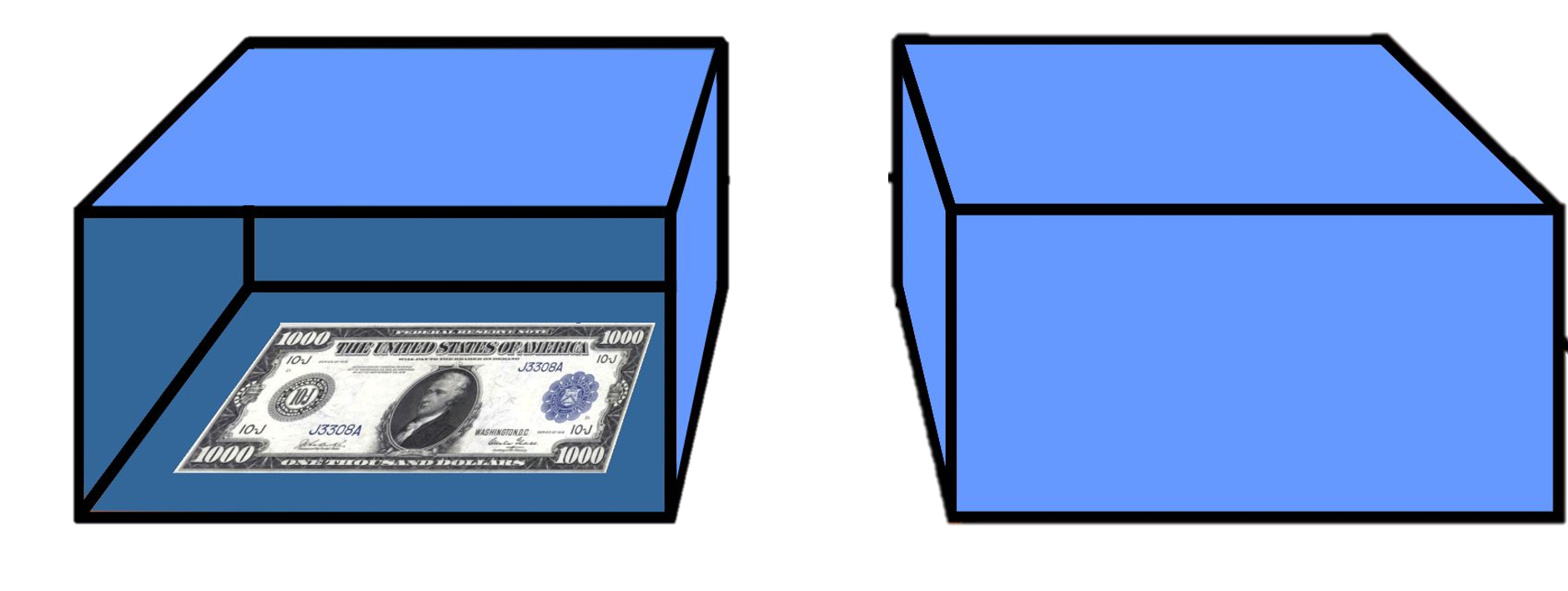… pour juger de ce que l'on doit faire pour obtenir un bien ou pour éviter un mal,
il ne faut pas seulement considérer le bien & le mal en soi,
mais aussi la probabilité qu'il arrive ou n'arrive pas;
& regarder géometriquement la proportion que toutes ces choses ont ensembles …

- Antoine Arnauld & Pierre Nicole's (1662, IV, 16) La logique, ou l'art de penser in the original French

… to judge what one ought to do to obtain a good or avoid an evil,
one must not only consider the good and the evil in itself,
but also the probability that it will or will not happen;
and view geometrically the proportion that all these things have together …
- Jeffrey's (1981, p. 473) translation

## Causal Decision Theory

1. Causal Decision Theory:
2. Causal Decision Theory relies on probabilities of conditionals instead of conditional probabilities
3. Under Causal Decision Theory, good decisions aim to produce (i.e. bring about) good outcomes
4. Expected utility tracks efficacy rather than auspiciousness
5. This may be contrasted with Evidential Decision Theory, under which good decisions are indicative of (i.e. provide evidence for) good outcomes

 Decision Matrix for Newcomb's Problem PREDICTION 1(Put nothing in Box 2) PREDICTION 2(Put \$1,000,000 in Box 2) φ1 (Take Box 2 only) \$0 (outcome o13) \$1,000,000 (outcome o15) φ2 (Take Box 1 and Box 2) \$1,000 (outcome o24) \$1,001,000 (outcome o26)

1. U(o13) = 0
2. U(o24) = 1,000
3. U(o15) = 1,000,000
4. U(o26) = 1,001,000

5. Let > denote a subjunctive connective
6. Let α denote a possible world
7. Let f denote the selection function operating on a suitable similarity metric of possible worlds
8. Let f(A, α) denote the selected world that differs minimally from the actual world in which B is evaluated

9. A > B is true in α if B is true in f(A, α)
10. A > B is false in α if B is false in f(A, α)
11. NOTE: Your action of φ1-ing or φ2-ing does not cause the prediction of the daemon predictor (PREDICTION 1 or PREDICTION 2), which happened in the past

12. According to the dominance principle:
13. The world has been partitioned into 2 with PREDICTION 1 (Put nothing in Box 2) and PREDICTION 2 (Put \$1,000,000 in Box 2)
14. In the 1st partition (PREDICTION 1), in which the daemon predictor puts nothing in Box 2, two-boxing (yielding outcome o24 and \$1,000) dominates one-boxing (yielding outcome o13 and \$0)
15. In the 2nd partition (PREDICTION 2), in which the daemon predictor puts \$1,000,000 in Box 2, two-boxing (yielding outcome o26 and \$1,001,000) dominates one-boxing (yielding outcome o15 and \$1,000,000)

16. The formula for computing the expected utility of an action becomes:
17. ### EU(φi) = ΣP(φi > oij) × u(oij)

18. Let s1 denote the state into which the world has been partitioned by PREDICTION 1
19. Let s2 denote the state into which the world has been partitioned by PREDICTION 2
20. Let p denote P(s1)
21. ∴ (1 - p) will denote P(s2)
22. ∴ P(φ1 > o13) = P(s1) = p
23. ∴ P(φ2 > o24) = P(s1) = p
24. ∴ P(φ1 > o15) = P(s2) = 1 - p
25. ∴ P(φ2 > o26) = P(s2) = 1 - p

26. EU(φ1) = p(0) + (1 - p)(1,000,000) = 1,000,000 - 1,000,000p
27. EU(φ2) = p(1,000) + (1 - p)(1,001,000) = 1,001,000 - 1,000,000p
28. EU(φ2) - EU(φ1) = 1,000

29. ∴ We arrive at RECOMMENDATION 2 under this version of causal decision theory:
30. Two-box, φ2, and take Box 1 and Box 2
31. RECOMMENDATION 2 is in accordance with the dominance principle